• 矢量求导的微分法则： 链式法则介绍核心原理矢量求导矩阵求导链式法则 介绍 这篇博文推导了矢量情形下， 标量函数矢量进行求导的微分法则，从定义出发推导了链式法则的形式。 核心原理 核心原理： 标量情形下， 由...
矢量求导的微分法则： 链式法则介绍核心原理矢量求导矩阵求导链式法则
介绍
这篇博文推导了矢量情形下， 标量函数对矢量进行求导的微分法则，从定义出发推导了链式法则的形式。
核心原理
核心原理：
标量情形下， 由中学的标量求导知识可知，忽略泰勒展开高次项，有： $\Delta f(x) = {f^{'}}(x) \Delta x$
即， 函数变化量 = 导数 * 变量变化量。
拓展到多变量情形下，显然有类似的：$\Delta f(x,y) = {f^{'}}(x)\Delta x + {f^{'}}(y)\Delta y$。
矢量求导
而一个矢量，可以看做多个标量的组合，如有矢量 $\mathbf{x}=\left[\begin{array}{l}{x_{1}} \\ {x_{2}}\end{array}\right]$
$f(\mathbf{x})$是以矢量 $\mathbf{x}$为变量的标量函数， 那么根据上面的知识，有:
$\Delta f(\mathbf{x}) = {f^{'}}(x_1)\Delta x_1 + {f^{'}}(x_2)\Delta x_2$
利用线性代数知识，可以将其写为矩阵形式：
$\Delta f(\mathbf{x}) = \left[\begin{array}{ll}{{f^{'}}(x_1)} & {{f^{'}}(x_2)}\end{array}\right] \times \left[\begin{array}{l}{\Delta x_{1}} \\ {\Delta x_{2}}\end{array}\right]$
其中，${f^{'}}(x_1)$是一个多变量函数对单变量求导的结果，也被称为偏微分， 可写为：
${{f^{'}}(x_1)}=\frac{{\partial f({\bf{x}})}}{{\partial {x_1}}}$
同理, ${{f^{'}}(x_2)}=\frac{{\partial f({\bf{x}})}}{{\partial {x_2}}}$
变化量的$\Delta$符号往往用$\mathbf{d}$代替，利用这些表示，式子可以改写为：
$\mathbf{d} f(\mathbf{x}) = \left[\begin{array}{ll}{\frac{{\partial f({\bf{x}})}}{{\partial {x_1}}}} & {\frac{{\partial f({\bf{x}})}}{{\partial {x_2}}}}\end{array}\right] \times \left[\begin{array}{l}{\mathbf{d}x_{1}} \\ {\mathbf{d} x_{2}}\end{array}\right]$.
注意到，根据矢量微分的定义，标量函数对一个矢量求导的结果可表示为：
$\nabla_{\mathbf{x}}f(\mathbf{x})= \left[\begin{array}{l}{\frac{{\partial f({\bf{x}})}}{{\partial {x_1}}}} \\ {\frac{{\partial f({\bf{x}})}}{{\partial {x_2}}}}\end{array}\right]$
上式也被称为函数$f(\mathbf{x})$对于$\mathbf{x}$的梯度。
利用这一定义，可以进一步的：
$\mathbf{d} f(\mathbf{x}) =\nabla_{\mathbf{x}}f(\mathbf{x})^T\mathbf{d} \mathbf{x}$.
其中，$\mathbf{d} \mathbf{x}=\left[\begin{array}{l}{\mathbf{d}x_{1}} \\ {\mathbf{d} x_{2}}\end{array}\right]$, 上式也被称为对矢量$\mathbf{x}$的全微分。
注意，为了便于理解，笔者以一个$2\times1$的矢量举例，但无疑，类似的过程可以推导出N维矢量的结论，从而统一为上式的表示。
矩阵求导
严格来说，矩阵也是矢量的一种。在许多时候，对于一个变量为$x\times y$的矩阵的求导问题，我们常用的处理方式是可以将其列化为$xy*1$的向量，再对其进行求导。为了便于理解，笔者同样以一个最简单的$2\times2$矩阵$\mathbf{X}$举例了：
$\mathbf{X}=\left[\begin{array}{ll}{x_1} & {x_3} \\ {x_2} & {x_4}\end{array}\right]$
根据定义（可以搜索维基百科），矩阵的求导（梯度）可以表示为：
$\nabla_{\mathbf{X}}f(\mathbf{X})=\left[\begin{array}{ll}{\frac{\partial f}{\partial x_{1}}} & {\frac{\partial f}{\partial x_{3}}} \\ {\frac{\partial f}{\partial x_{2}}} & {\frac{\partial f}{\partial x_{4}}}\end{array}\right]$.
而如果对$\mathbf{X}$进行列化的话，求得的向量梯度中包含的元素，刚好对应了矩阵梯度的元素。 也就是说，一种解决的思路是：

将矩阵变量列化为向量
利用向量求导的结论，求出梯度
将向量梯度矩阵化，就是所求的矩阵梯度。

这个方法非常有效，但有些时候，矩阵变量不容易向量化，如目标函数是矩阵的逆的时候。那么，我们可以按照上节的写法，推广到矩阵的形式，有：
$\mathbf{d} f(\mathbf{X}) =\mathrm{tr}(\nabla_{\mathbf{X}}f(\mathbf{X})^T\mathbf{d} \mathbf{X})$.
这个读者可以自己推导一下，和上节的原理一致，由于线性代数的原因，在矩阵情况下会多一个tr(trace)。
链式法则
先讨论实数的情况：
标量情形下，我们知道，若$f(x) =f(g(x))$, 那么$f^{'}(x)=f^{'}(g)\times g^{'}(x)$.
多维情况下，根据之前的类似思想，也可以推导结果，但这里我们使用上面讲述的方法
假设标量函数$f(x)$, 中间变量矢量$\mathbf{g}$, 变量$\mathbf{x}$.
根据上面推导：
$\mathbf{d} f(\mathbf{g}) =\nabla_{\mathbf{g}}f(\mathbf{g})^T\mathbf{d} \mathbf{g}$.
$\mathbf{d} \mathbf{g} = \nabla_{\mathbf{x^T}}\mathbf{g}(\mathbf{x})\mathbf{d} \mathbf{x}$
(之前没有讨论矢量对矢量微分的情形，但显然很容易从标量函数情形推广)
因此有：
$\mathbf{d} f(\mathbf{g}) =\nabla_{\mathbf{g}}f(\mathbf{g})^T \nabla_{\mathbf{x^T}}\mathbf{g}(\mathbf{x})\mathbf{d} \mathbf{x}$.
可得：
$\nabla_{\mathbf{x}}f(\mathbf{x}) = (\nabla_{\mathbf{g}}f(\mathbf{g})^T \nabla_{\mathbf{x^T}}\mathbf{g}(\mathbf{x}))^T= \nabla_{\mathbf{x}}\mathbf{g}^T\times \nabla_{\mathbf{g}}f(\mathbf{g})$.
最后，总结下多种情形链式法则的结论，推导过程均可由上面过程拓展：

标量 $f$, 矢量$\mathbf{g}$, 标量$x$
$\nabla_{x}f(x) =\frac{{\partial f}}{{\partial x}}=\frac{{\partial f}}{{\partial {\mathbf{g}^T}}} \times \frac{{\partial \mathbf{g}}}{{\partial x}}$
*标量 $f$, 矢量$g$, 矢量$x$
$\frac{{\partial f}}{{\partial \mathbf{x}}}=\frac{{\partial \mathbf{g}^T}}{{\partial \mathbf{x}}}\times \frac{{\partial f}}{{\partial {\mathbf{g}}}}$
*矢量 $f$, 矢量$g$, 矢量$x$
$\frac{{\partial \mathbf{f}}}{{\partial \mathbf{x}}}=\frac{{\partial \mathbf{g}^T}}{{\partial \mathbf{x}}}\times \frac{{\partial \mathbf{f}}}{{\partial {\mathbf{g}}}}$
*标量 $f$, 矩阵$g$, 标量$x$
$\frac{{\partial f}}{{\partial x}}= \mathrm{tr}(\frac{{\partial f}}{{\partial {\mathbf{g}^T}}} \frac{{\partial \mathbf{g}}}{{\partial x}})$


展开全文• 矢量求导之位移与速度及加速度
矢量求导之位移与速度及加速度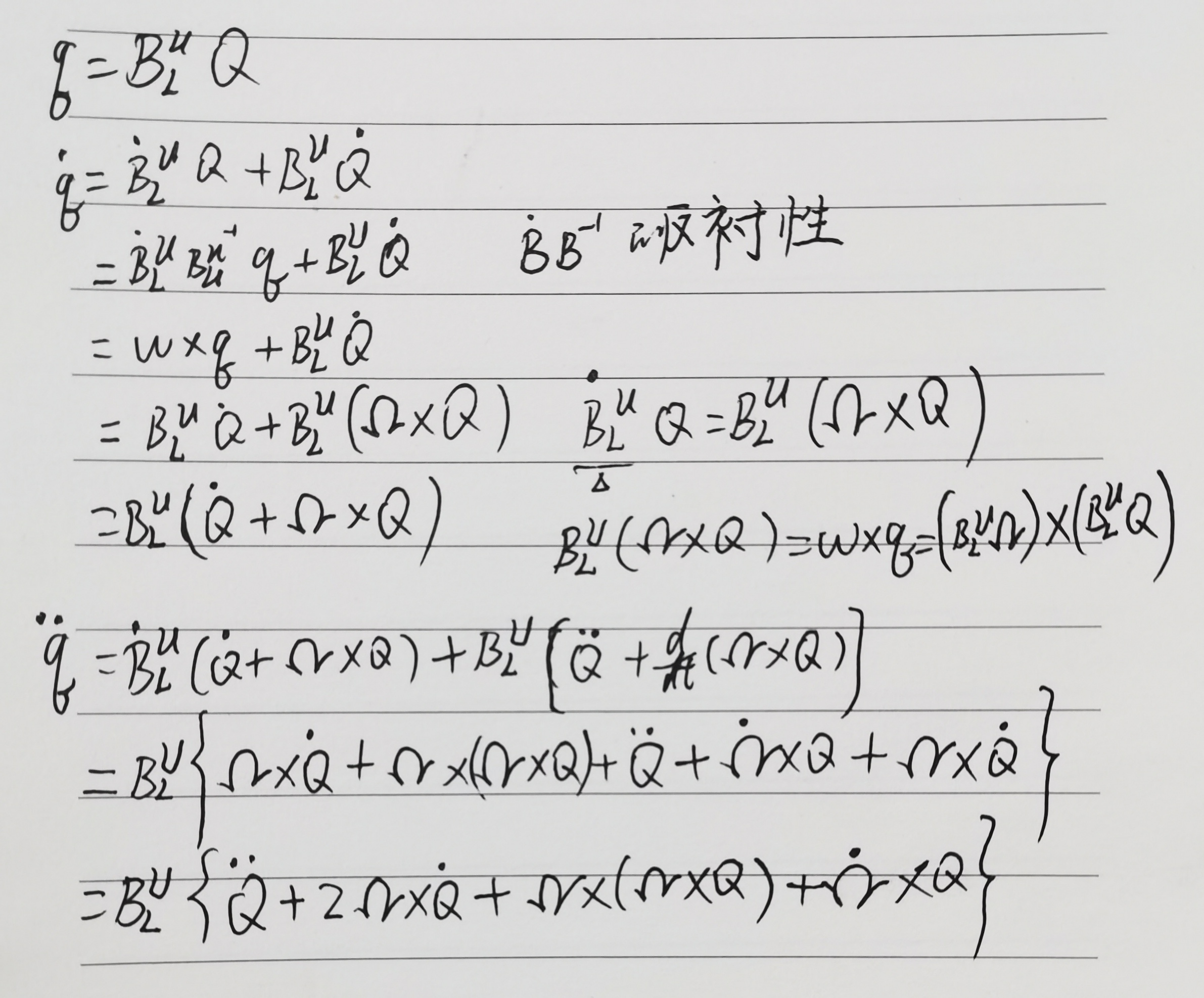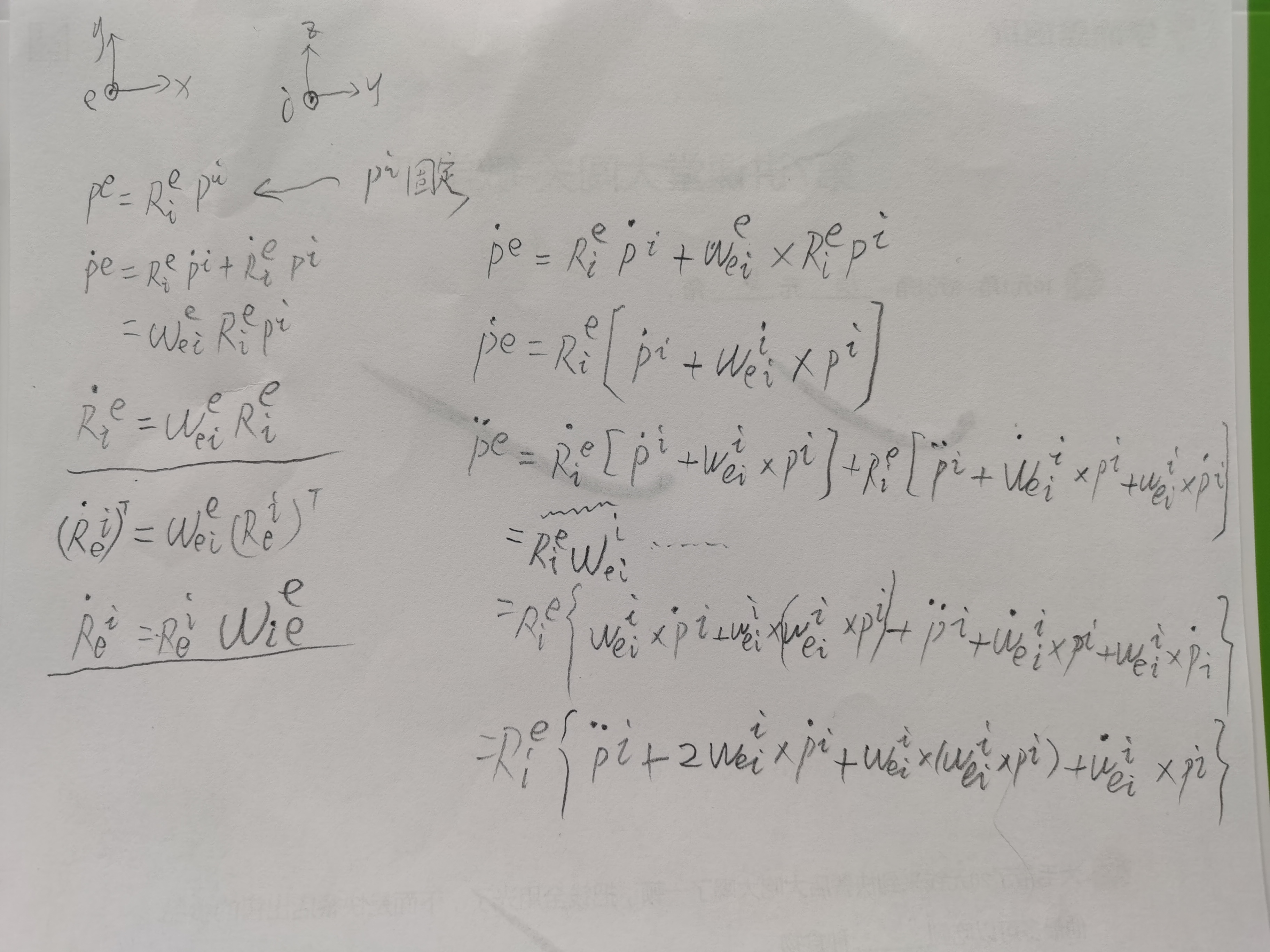展开全文• 是KX1的矢量函数： f ( x ) = ( f 1 ( x ) f 2 ( x ) ⋮ f K ( x ) ) f(x)=\left(\begin{array}{c}{f_{1}(x)} \\ {f_{2}(x)} \\ {\vdots} \\ {f_{K}(x)}\end{array}\right) f ( x ) = ⎝ ⎜ ⎜ ⎜ ⎛ ​ f 1 ​ ( ...
Definition D.l：
设:$f(x)$是标量,
$x=\left(x_{1} \ldots x_{N}\right)^{T}$
,那么：
$\frac{\partial f(x)}{\partial x}=\left(\begin{array}{c}{\frac{\partial f(x)}{\partial x_{1}}} \\ {\frac{\partial f(x)}{\partial x_{2}}} \\ {\vdots} \\ {\frac{\partial f(x)}{\partial x_{N}}}\end{array}\right)$
$\left(\frac{\partial f(x)}{\partial x}\right)^{T}=\frac{\partial f(x)}{\partial x^{T}}=\left(\begin{array}{cc}{\frac{\partial f(x)}{\partial x_{1}}} & {\frac{\partial f(x)}{\partial x_{2}}}\end{array} \ldots \frac{\partial f(x)}{\partial x_{N}}\right)$
--------------------------------------------------------------------------------------
Definition D.2
假设$f(x)$二阶可导：
$\frac{\partial^{2} f(x)}{\partial x \partial x^{T}}=\left(\begin{array}{cccc}{\frac{\partial^{2} f(x)}{\partial x_{1}^{2}}} & {\frac{\partial^{2} f(x)}{\partial x_{1} \partial x_{2}}} & {\dots} & {\frac{\partial^{2} f(x)}{\partial x_{1} \partial x_{N}}} \\ {\frac{\partial^{2} f(x)}{\partial x_{2} \partial x_{1}}} & {\frac{\partial^{2} f(x)}{\partial x_{2}^{2}}} & {} & {\vdots} \\ {\vdots} & {} & {\ddots} & {\vdots} \\ {\frac{\partial^{2} f(x)}{\partial x_{N} \partial x_{1}}} & {\cdots} & {\cdots} & {\frac{\partial^{2} f(x)}{\partial x_{N}^{2}}}\end{array}\right)$
在假设
$\partial^{2} f(x) / \partial x_{p} \partial x_{q}=\partial^{2} f(x) / \partial x_{q} \partial x_{p}$成立的情况下，
该Hessian矩阵对称。
--------------------------------------------------------------------------------------
Dehition D3：
假设$f(x)$是KX1的矢量函数：
$f(x)=\left(\begin{array}{c}{f_{1}(x)} \\ {f_{2}(x)} \\ {\vdots} \\ {f_{K}(x)}\end{array}\right)$
$x=\left(x_{1} \ldots x_{N}\right)^{T}$
则$f(x)$的Jacobian矩阵是：
$\frac{\partial f(x)}{\partial x^{T}}=\left(\begin{array}{cccc}{\frac{\partial f_{1}(x)}{\partial x_{1}}} & {\frac{\partial f_{1}(x)}{\partial x_{2}}} & {\dots} & {\frac{\partial f_{1}(x)}{\partial x_{L}}} \\ {\frac{\partial f_{2}(x)}{\partial x_{1}}} & {\frac{\partial f_{2}(x)}{\partial x_{2}}} & {\dots} & {\frac{\partial f_{2}(x)}{\partial x_{L}}} \\ {\vdots} & {\vdots} & {} & {\vdots} \\ {\frac{\partial f_{K}(x)}{\partial x_{1}}} & {\frac{\partial f_{K}(x)}{\partial x_{2}}} & {\dots} & {\frac{\partial f_{K}(x)}{\partial x_{L}}}\end{array}\right)$
该Jacobian矩阵的转置是：
$\left(\frac{\partial f(x)}{\partial x^{T}}\right)^{T}=\frac{\partial f^{T}(x)}{\partial x}$
--------------------------------------------------------------------------------------
定理4我感觉是十分显而易见，所以就没有转载。
Reference:
APPENDIX D VECTOR AND MATRIX DIFFERENTIATION


展开全文• 矢量对矢量求导的定义 链式求导法则 链式法则的应用1：Jacobi矩阵的逆 链式法则的应用2：标量矩阵的求导
矢量对矢量求导的定义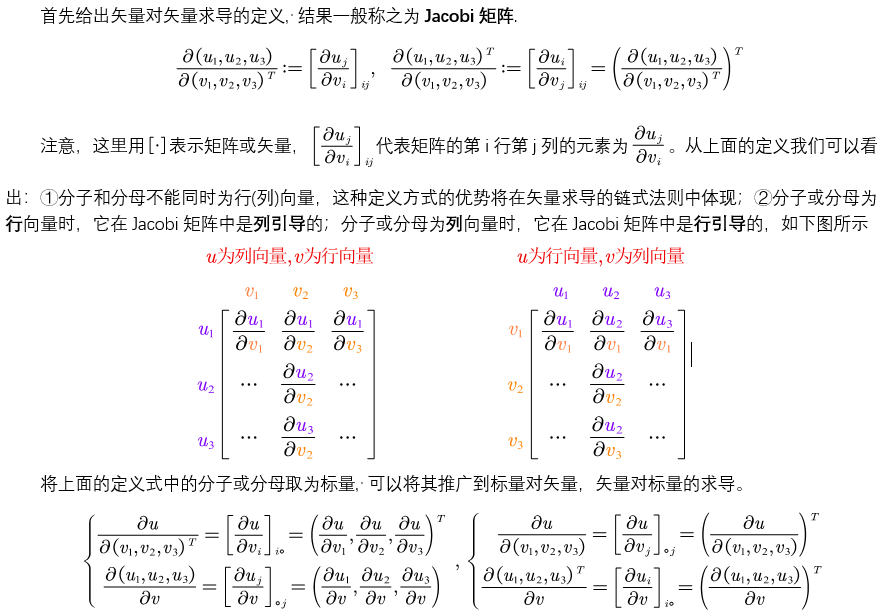链式求导法则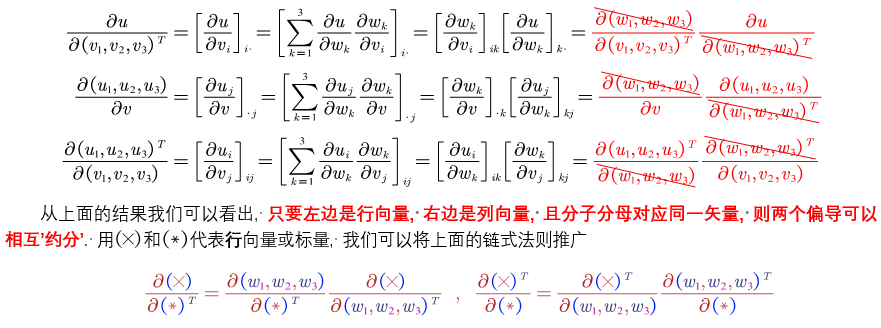链式法则的应用1：Jacobi矩阵的逆链式法则的应用2：标量对矩阵的求导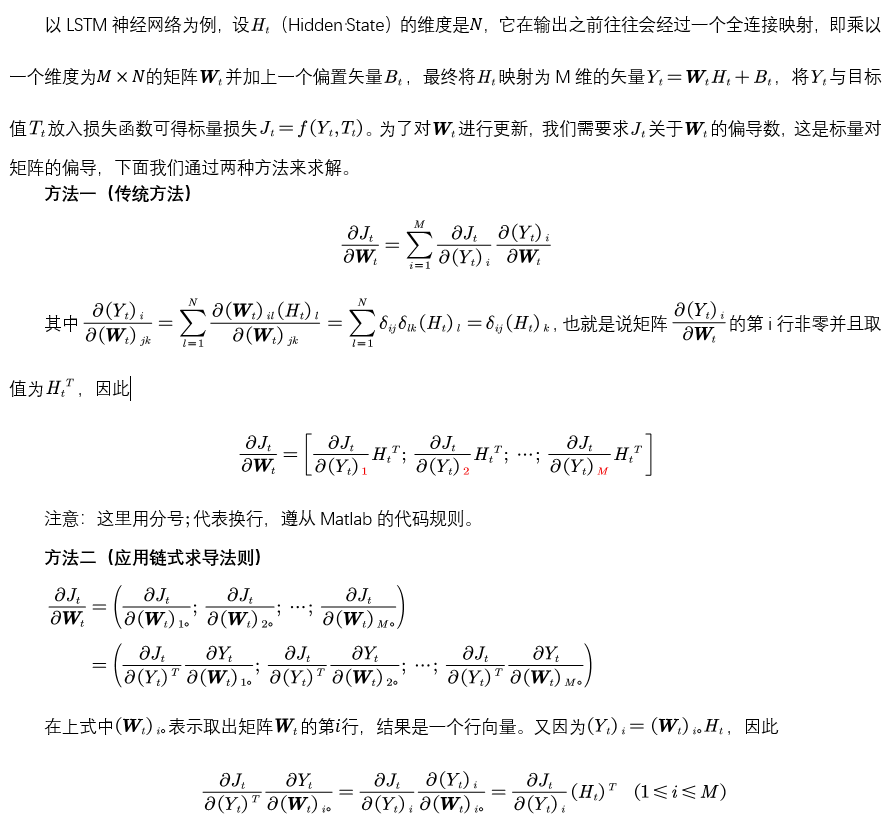展开全文线性代数 矩阵 神经网络 机器学习 深度学习
• 向量向量求导 向量向量求导法则，按照分母为主导，参考：向量向量求导法则 文件是在网上找的链接，如有侵权请联系博主删除。
• 行向量元素求导列向量元素求导矩阵元素求导元素对矢量求导元素对矢量求导，相当于元素和矢量里的每一个元素求一次导，结果的形式和矩阵的形式相同。元素行向量求导元素列向量求导元素矩阵求导矢量对矢量...矩阵运算 数学
• 1.标量向量求导 标量可以直接向量求导 import torch p = torch.tensor([2.0, 3.0, 5.0], requires_grad=True) q = p*2+1 z = torch.mean(q) z.backward() print(p.grad) print(p.grad*p) 结果： tensor([0.6667...
• 本文标量、矢量、矩阵的求导概念和公式做简单归纳梳理，目的是帮助理解和记忆，不涉及具体推导过程。线性代数 矩阵
• 谁求导数，就以谁（分母）作为主序，得出结果。比如这里x是列向量，求Ax关于x求导数，那么x的每个分量分别求偏导数(写成一行)，然后整理排成一列（同x一样是列向量）。 同理有 关于x的转置x.T求导数，...
• https://www.cnblogs.com/pinard/p/10773942.html
• ## 函数对向量的求导

千次阅读 2020-04-17 17:37:53
我们一般函数求导，是单变量求导，但是在机器学习中，会遇到多元函数向量求导的情况，比如： f(w⃗)=12∣∣w⃗∣∣2 f(\vec{w})=\frac{1}{2}||\vec{w}||^2f(w)=21​∣∣w∣∣2其中，w⃗=(w1,w2,⋯ ,wn)\vec{w...
• 在这个式子中用到了标量函数向量求导，也就是： ∂ ∂ w ( 1 2 ∥ w ∥ 2 ) = w （ 公 式 3 ） ∂ ∂ w ( 1 2 ∥ w ∥ 2 ) = w （ 公 式 3 ） \begin{equation*} \frac{\partial }{\partial w}(\frac{1}{2}{\...SVM 支持向量机
• ## 对向量、方阵的求导

万次阅读 多人点赞 2016-07-05 11:04:45
平时都是一个数求导，那向量求导呢？看下面的例子：  假设有下面这样的矩阵A和向量X    我们很容易求出    现在另 y = A·X，那矩阵y向量x求偏导的结果是多少？  我们来分析一下，既然是矩阵y向量x...
• matlab求导代码介绍 仿真代码，用于使用辅助矢量滤波的概念验证自适应扩频水下通信。 包括由东北航空的P. Qarabaqi和M. Stojanovic开发的，用于生成随时间变化的频道的实现方案，以及由SUNY Buffalo的P. Markopoulos...
• ## 向量的求导

万次阅读 多人点赞 2018-05-04 20:55:17
向量或者矩阵元素的求导很简单元素想两矩阵求导依然简单行列与列行 注意分母的对应行行 列列矩阵行向量以及列向量m*np矩阵求导先将X看成行向量，再将Y看成列向量参考链接：...
• 为什么微小扰动进行求导？ 链式求导是一个很常用、很简单的模型，但是为什么用这个模型呢？在SLAM十四讲中并没有这个问题进行阐述。 这与非线性优化的方法有关，首先对于一个最小二乘问题： min⁡x12∥f(x)∥22 ...
• 1. 向量、矩阵元素求导 2 1.1 行向量元素求导 2 1.2 列向量元素求导 2 1.3 矩阵元素求导 2 2. 元素向量、矩阵求导 3 2.1 元素行向量求导 3 2.2 元素列向量求导 3 2.3 元素矩阵求导 3 3. 向量向量...
• 最近在网上看到一篇很好的文章，清楚地讲解了机器学习中函数矩阵的求导公式和法则。在这里转载一下，大家可以去作者的这个知乎专栏看到这篇文章： https://zhuanlan.zhihu.com/p/25063314 ...机器学习
• 目录 前言 一、公式推导 1.1 将评分函数表达为矩阵形式 1.2 标量列向量求导规则 1.3 单个样本权重求导到全训练集 二、实现代码 前言 矩阵矩阵求导法则： 从上面公式可以看出，矩阵矩阵求导，会导致维度倍增...深度学习
• ## 向量求导公式

千次阅读 2016-12-15 16:00:01
最近在看吴恩达的视频啊、LDA啊、PCA啊，觉得很有必要将向量求导公式复习一下，要不感觉算的时候怪怪的~
• 反向传播算法 不失一般性， 第 层中的参数 W ( l ) \boldsymbol{W}^{(l)} W(l) 和 b ( l ) \boldsymbol{b}^{(l)} b(l) 计算偏导数． 这里使用向量或矩阵来表示多变量函数的偏导数， 并使用分子布局表示， 根据...深度学习 线性代数
• 1（1）标量标量求导 （2）向量标量求导 （3） 矩阵标量求导 2（1）标量向量求导 （2）向量向量求导 （3） 矩阵向量求导 3（1）标量矩阵求导 （3） 矩阵矩阵求导 附上matlab代码，可...
• ## 常用的向量矩阵求导公式

万次阅读 多人点赞 2016-10-14 14:29:06
总结下数理推导中常用的向量矩阵求导公式，方便以后查询。矩阵
• 本质上来说，一个函数(行)向量求导，本质上还是单独为向量的每个元素进行求导的。 比如 R n → R R^n \to R 的函数 f ( a ⃗  ) f(\vec a) ，则其导数【即梯度】为 ∇ f = [ ∂ f ∂ a 1 , ∂ f ∂ a 1 , ⋯ ...数学 线性代数
• 文章目录矩阵，向量求导(Matrix calculus)0.约定1.目标2.完整的求导表格完整表格1.布局说明分子布局（Numerator-layout）：分母布局（Denominator-layout）：2.举个例子分子布局例子分母布局例子3.表格细化1.Vector-...
• 构建深度学习模型的基本流程就是：搭建计算图，求得损失函数，然后计算损失函数模型参数的导数，再利用梯度下降法等方法来更新参数。 搭建计算图的过程，称为“正向传播”，这个是需要我们自己动手的，因为我们...
• 包括一些基本的矩阵向量求导 本文的目标读者是想快速掌握矩阵、向量求导法则的学习者，主要面向矩阵、向量求导在机器学习中的应用。因此，本教程而非一 份严格的数学教材，而是希望帮助读者尽快熟悉相关的求导方法并...pytorch...